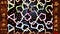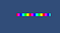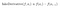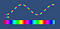# Drawing Dynamic Lasers Curved

## Making fun with meshes

Lasers are cool. And drawing a laser in a straight line is easy enough: just plop down a sprite, maybe repeat it a few times, maybe stretch it.

But what if you want curved lasers? And what if you want a hundred of them updating at 120 frames per second?

When I first tried to answer this question, I could not find any resources on how to do this in a reasonably noob-friendly way. So I learned how meshes work, and here I am writing the resource myself.

Luckily, this problem is a lot harder to work through than it is to solve. And no shaders are required!

While this article deals with Unity, it should be possible to apply this logic in a similar way to any other graphics engine.This article is way too short to teach you how to do this, but we’ll get partway there.

All the code for this post can be found on this Github repo licensed under CC0 (effectively public domain).

# Manual Mesh Generation

The main task here is to create a mesh, ie. a skeleton, in the correct shape. The two key components to a mesh are triangles and vertices. Each triangle is a list of three vertices. For each triangle, the renderer will use the three vertices to interpolate values for each of the pixels in between them, and then draw the pixels. The vertices can carry almost any information, but there are two things that are usually necessary in all situations: location and UV. Location indicates where the vertex is in world-space, and UV indicates where the vertex is on the material texture used to fill the mesh.

The code for generating the mesh itself is mostly boilerplate; the complex work is in assigning vertices. Let’s proceed through the steps:

`public static class MeshUtils {    private static readonly VertexAttributeDescriptor[] layout = {        new VertexAttributeDescriptor(VertexAttribute.Position, VertexAttributeFormat.Float32, 3),         new VertexAttributeDescriptor(VertexAttribute.TexCoord0, VertexAttributeFormat.Float32, 2),     };    [System.Runtime.InteropServices.StructLayout(System.Runtime.InteropServices.LayoutKind.Sequential)]    public struct VertexData {        public Vector3 loc;        public Vector2 uv;    }`

The first thing we do is declare what kind of information we are going to store in our vertices. A Vector3 for location and a Vector2 for UV is a minimal setup. We will use the VertexData struct to store our data, and we will pass the layout object to the mesh so it knows how to read our data.

Note that, even though location and UV are the same “type”, we have to give them different VertexAttributes in the layout object. These VertexAttribute correspond to “semantics” in HLSL, which basically indicate the use of a variable. `Position` and `TexCoord0` are the standard semantics for the location and UV vectors in shaders, so we mark them as such here.

`public static int[] WHTris(int h, int w) {        int vw = w + 1;        int[] tris = new int[2 * h * w * 3];        for (int ih = 0; ih < h; ++ih) {            for (int iw = 0; iw < w; ++iw) {                int it = 2 * (w * ih + iw) * 3;                int iv = ih * vw + iw;                tris[it + 0] = iv;                tris[it + 1] = iv + vw + 1;                tris[it + 2] = iv + 1;                                tris[it + 3] = iv + vw + 1;                tris[it + 4] = iv;                tris[it + 5] = iv + vw;            }        }        return tris;    }`

Then, we need a function that generates triangles in the required layout. We are thinking of lasers in terms of “a long rectangle that gets deformed”, so we can use this basic triangle generation function that creates triangles for a rectangular shape. `2*h*w` is the number of triangles for a rectangle that is `w` by `h` units, and the image below shows how the loop code is determined.

`public static (Mesh, NativeArray<VertexData>) CreateMesh(int h, int w) {        int numVerts = (h + 1) * (w + 1);        int[] tris = WHTris(h, w);        var mesh = new Mesh();        mesh.SetVertexBufferParams(numVerts, layout);        mesh.bounds = new Bounds(Vector3.zero, Vector3.one * 100f);        mesh.triangles = tris;        return (mesh, new NativeArray<VertexData>(numVerts, Allocator.Persistent));    }`

Finally, we can put the steps together to create a mesh. The most important thing to note here is that a rectangle mesh that is 2 by 2 units has 9 vertices. This is because we require a vertex at the end of the rectangle in order to draw the last part of the rectangle. A 1 by 1 rectangle (ie. a square) has 4 vertices, one for each corner. This is important to remember.

You’ll also notice that I assign an arbitrary large value to `mesh.bounds`-- I could not find a situation where this affects anything.

If you haven’t seen `NativeArray` before, it's a type that Unity uses to pass information between managed and unmanaged memory. It's an array in proper sequential malloc style, and you can treat it as a pointer to make computations over it faster. We need to store our vertices in a NativeArray in order to efficiently pass them to the mesh internal code.

# Drawing a Mesh

Now, we can use our rectangle mesh to manually draw a shape to the screen. First, though, the boilerplate:

`public class BasicLaser : MonoBehaviour {    private Mesh mesh;    private NativeArray<VertexData> verts;    private MeshFilter mf;    private MeshRenderer mr;    private MaterialPropertyBlock pb;    public Sprite sprite;    private int w = 100;    private int h = 1;    private void Awake() {        pb = new MaterialPropertyBlock();        pb.SetTexture(Shader.PropertyToID("_MainTex"), sprite.texture);        mr = GetComponent<MeshRenderer>();        mf = GetComponent<MeshFilter>();        (mesh, verts) = MeshUtils.CreateMesh(h, w);        mf.mesh = mesh;        Draw();        Commit();    }​    private void Update() {        Draw();        Commit();    }​    private void Commit() {        mesh.SetVertexBufferData(verts, 0, 0, verts.Length);        mr.SetPropertyBlock(pb);    }​    private void OnDestroy() {        verts.Dispose();    }}`

Our GameObject will require a MeshRenderer and MeshFilter component. The MeshRenderer can be populated with a default material using a default sprite shader, and we populate the MeshFilter in code. Since we’re using MeshRenderer, we need to use a MaterialPropertyBlock to provide the sprite texture to the shader. `_MainTex` is the default name for the main texture in almost all Unity shaders.

We’ll write some Draw method that fills the vertices with the correct values. Then, we have a simple `Commit` method which writes the vertices to the mesh.

Note that we must `Dispose` of the vertex array manually, since it is a NativeArray.

To ensure this works, here’s a basic Draw method. It draws the laser from left to right. Note that it handles UVs manually, so the rendered laser will not have the correct scale when compared to the sprite. We’ll fix this in our generalized method.

`private void Draw() {        int vw = w + 1;        for (int iw = 0; iw <= w; ++iw) {            for (int ih = 0; ih <= h; ++ih) {                var v = verts;                v.loc.x = iw / 10.0f;                v.loc.y = ih;                v.uv.x = iw / 40.0f;                v.uv.y = ih;                verts[iw + ih * vw] = v;            }        }    }`We have now replicated dropping a sprite on the screen.

# Getting Real With Equations

It’s easiest to think about a laser as a rectangle that is many units long and one unit high, which follows some parametric equation `f(t)=<x,y>`. In order to draw a laser around an equation, we can sample points on the equation and draw axes on the normal.

However, since we’ll be sampling the equation discretely and without a derivative function, we need a substitute. I find that the most consistent way to deal with this is to use the familiar approximation:This is not latex, it is a screenshot of latex in my Markdown editor. Still waiting for Medium latex support.

In other words, the derivative to the equation at point `#i` is determined by the difference between point `#i-1` and point `#i`. I have omitted the denominator because we only need the unit derivative vector, so the magnitude does not matter. For convenience, we should set the derivative at point 0 to the same as the derivative at point 1.

If we have a unit derivative vector `d`, and the high and low points are `sh` away from the center line, then we have the following relations:

`lower-point = center + sh * <d.y, -d.x>` (ie. `center + sh * rot(d, -90)`)

`higher-point = center + sh * <-d.y, d.x>` (ie. `center + sh * rot(d, 90)`)

Note that the low point is `uv[i]` and the high point is `uv[i + w + 1]`.

With this knowledge, we can proceed to write our proper draw function. For convenience, I’ve done this with pointers, although you could do it “safely” by copying and reassigning struct values.

`private unsafe void Draw() {        Func<float, float, Vector2> drawFunc = (dt, lt) => new Vector2(dt, Mathf.Sin(dt + lt));        int vw = w + 1;        float sh = spriteBounds.y / 2f;        var vertsPtr = (VertexData*)verts.GetUnsafePtr();                vertsPtr.uv.x = vertsPtr[vw].uv.x = 0f;                 Vector2 loc = drawFunc(0f, lifetime);        float drawtime = updateStagger;        Vector2 nextLoc = drawFunc(drawtime, lifetime);        Vector2 delta = nextLoc - loc;        Vector2 unit_d = delta.normalized;        vertsPtr.loc.x = loc.x + sh * unit_d.y;        vertsPtr.loc.y = loc.y + sh * -unit_d.x;        vertsPtr[vw].loc.x = loc.x + sh * -unit_d.y;        vertsPtr[vw].loc.y = loc.y + sh * unit_d.x;        vertsPtr.uv.y = 0;        vertsPtr[vw].uv.y = 1;        for (int iw = 1; iw < vw; ++iw) {            vertsPtr[iw].uv.x = vertsPtr[iw + vw].uv.x = vertsPtr[iw - 1].uv.x + delta.magnitude / spriteBounds.x;            vertsPtr[iw].loc.x = loc.x + sh * unit_d.y;            vertsPtr[iw].loc.y = loc.y + sh * -unit_d.x;            vertsPtr[iw + vw].loc.x = loc.x + sh * -unit_d.y;            vertsPtr[iw + vw].loc.y = loc.y + sh * unit_d.x;            vertsPtr[iw].uv.y = 0;            vertsPtr[iw + vw].uv.y = 1;                        drawtime += updateStagger;            loc = nextLoc;            nextLoc = drawFunc(drawtime, lifetime);            delta = nextLoc - loc;            unit_d = delta.normalized;        }    }`

The function I created is a sine-wave that modulates as the laser gets older. (You could slot in basically any function here without an issue.) When thinking about laser parametric equations, there are two forms of time: the time along the drawing path of the laser, and the lifetime of the laser itself. If the draw-time is fixed, then the laser is a single point that moves. If the lifetime is fixed, then the laser is a curved line that does not change.

Each point is separated by a draw-time difference of `updateStagger`. When this is lower, points will be sampled more densely and the laser will be smoother.

The main loop of this code involves calculating the next point, getting the delta, and then setting the points on the laser rectangle according to the relations we calculated earlier. In addition, we set the UV values of each of the vertices. The lower points should have a y-UV of 0 and the higher points should have a y-UV of 1; this is because we draw the laser horizontally. The x-UVs are determined by adjusting the delta in accordance with the size of the sprite; this makes the sprite tile across the laser evenly. In most cases, the x-UVs will go much higher than 1; if we set our sprite tiling to Repeat in the U direction, then the laser texture will repeat when this occurs.The sprite is now automatically set to its actual size. Also, the laser moves.

# Wrapping Up

It’s not difficult to optimize the code above to make fast, efficient, curving lasers. And it’s not difficult to extend this concept to making similar complex laser-like objects. For example, lasers that move across the screen, like actual snakes.

Thanks for reading, and good luck with your lasers!

Again, all the code for this post can be found on this Github repo licensed under CC0.

Software engineer, epic gamer, and Touhou developer. All my writing is licensed under CC BY-SA 4.0 unless stated otherwise.

## More from Bagoum

Software engineer, epic gamer, and Touhou developer. All my writing is licensed under CC BY-SA 4.0 unless stated otherwise.